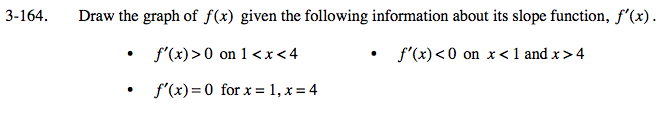### Home > CALC > Chapter Ch3 > Lesson 3.4.2 > Problem3-164

3-164.Notice that the clues describe f '(x), yet you are asked to sketch f(x).

f '(x) > 0 on 1 < x < 4
Translate: f(x) is increasing between x = 1 and x = 4.

f '(x) < 0 on x < 1 and x > 4
Translate: f(x) is decreasing before x = 1 and after x = 4.

f '(x) = 0 for x = 1, x = 4
Translate: x has reached a local maximum or local minimum at x = 1 and x = 4.